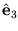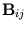## The General Analytical Rebridging Algorithm

The general analytical rebridging algorithm is a set of subroutines capable of solving the rebridging problem in molecular simulations. It is freely distributed under the GNU Public License.

# Description

The general analytical rebridging algorithm solves for solutions that direct a linear segment of the molecule towards a fixed end. It conserves the bond lengths and bond angles. By defining the appropriate rigid units, the user can choose to conserve some torsional angles, e.g., those for non-bonds, as well. It can be applied to cause a local conformational change of the internal part of a molecule. It can also be used to graft'' a molecule onto another molecule. The algorithm accommodates a general backbone geometry, from simple poly-ethylene to peptides and proteins. Although the algorithm focuses on solving the configuration of the backbone, side chains with free ends are allowed.

Consider a arbitrary segment of the molecular backbone shown in Fig. 1. The bonds shown in bold lines are chosen to be torsionally constrained. Given the the two bonds u1, u6 in the 3-d space, the algorithm finds all the solutions that re-insert in a valid way the backbone units within the dotted region. The variables to be solved are the six torsional anglesii = 1,..., 6. The number of variables is six, because the rebridging solution enforces six geometrical constraints towards the end.The general analytical rebridging algorithm is written in ANSI C (download do_rebridge_1.1.3.tar.gz, last updated 9 January 2006). An older version is still available (download do_rebridge_1.0.tar.gz, last updated 12 December 2000). The function to be called is do_rebridge. To make use of the subroutine
• Uncompress the file with gunzip do_rebridge.tar.gz
• Extract the file with tar -xvf do_rebridge.tar
• Copy all of the extracted files to your source directory
• Call the do_rebridge subroutine from your code

We ask that you cite our paper  in any publications that result from the use of the do_rebridge subroutine.

# Preparing the data input

The rebridging algorithm asks the user to input a set of parameters that describe the backbone geometry. These parameters can be calculated from a current valid configuration.

We denote ûi, i = 1,..., 6, as the unit axis of the ith torsional bond. Let ri be the 3-d position of the atom that ends the ith torsional bond. The idea is to construct a closed loop consisting of seven joints with u1u2,.., u7 and of seven links with vectors a1,a2, ..., a7, as shown in Fig. 2.Each link is perpendicular to the two adjacent joint vectors. How the links are calculated is given below. For i = 1,..., 5, calculate the following parameters:
1. Find the shortest vector, ai, that connects the lines containing the two consecutive torsional bonds ûi and ûi + 1. Choose the direction for ai so that it goes from ûi to ûi + 1. Let ai be the length of ai. Define âi as the unit axis of ai. If the lines containing ûi and ûi + 1 intersect with each other, ai = 0 and ai is a null vector. In this case, we choose âi as the unit axis perpendicular to both ûi and ûi + 1 and passing through the intersection. Choose the direction of âi so that it is parallel with ûix ûi + 1.
2. Calculatei, the twist'' angle, defined by ûi, âi, and ûi + 1. The twist'' anglei is zero when ûi and ûi + 1 are parallel to each other. In the ai = 0 case, the âi chosen in the previous step makesi always positive.
3. Find the shortest vector connecting ri and the line containing âi, and calculate the projection of this vector on ûi. This projection is called Si.
4. Find the shortest vector connecting ri + 1 and the line containing âi, and calculate the projection of this vector on - ûi + 1. This projection is called Ti.
The parameters extracted from the above calculations are ai,i, Si, and Ti, where i = 1,..., 5. Other parameters such as a7 are calculated by the algorithm automatically. Finally, the vectors û1, r1, û6, and r6 that specify the end constraints must be supplied.

# Function call and returned values

The arguments to be passed to do_rebridge are û1, r1, û6, r6, ai,i, Si, and Ti, i = 1,..., 5. They are shown in do_rebridge.c as follows

void do_rebridge(double uv1, double uv6,
double rv1, double rv6,
double a_in, double alpha_in,
double S_in, double T_in,
int *n_roots,
double **uv_solve[MAXROOTS], double **rv_solve[MAXROOTS])

Here MAXROOTS is 16, the maximum number of possible solutions. The first and second arguments pass the 3-d components of the unit axes û1 and û6. The third and fourth arguments pass the 3-d components of r1 and r6. In these arguments the subscript of the arrays goes from 0 to 2. The fifth to eighth arguments pass ai,i, Si, and Ti, where i = 1,..., 5. Note that in these arguments the subscript goes from 1 to 5. That is, a_in stores a1, S_in stores S5, and so on. The argument *n_roots returns the number of solutions found. It is always an even number between 0 and 16. The 3-dimensional array **uv_solve[MAXROOTS] returns ûi, i = 1,..., 6, for each solution. The 3-dimensional rray **rv_solve[MAXROOTS] returns ri, i = 1,..., 6, for each solution. Both arrays have the dimensions of 16 x 8 x 3 and have the same ranges. Take uv_solve[i][j][k] as an example, the valid ranges for the i, j, and k are 0,...,*n_roots-1, 1,..., 6, and 0,..., 2, respectively. The uv_solve[i] and rv_solve[i] vectors determine the positions of the five rebridged units, as determined in the ith solution of the rebridging equations.

A minimal example of a call to do_rebridge has been graciously provided by Stepan Ruzicka (stepan.ruzicka@epfl.ch). It can be found here (download example.tar.gz, last updated 4 August 2017).

In actual Monte Carlo simulations, each solution must be weighted by a associated Jacobian so as to account for the non-uniform distribution of the solutions in the torsional angle space. The Jacobian J takes this form:Hereis the z-axis of the reference coordinate. The determinant of the 5x5 matrix B can be evaluated by the function double mtx_det(double **a, int n) in mtxinv.c. The first argument, double **a, passes the elements of B, and the second argument n passes the size of the matrix, which is 5 in this case. The subscripts of the 2-dimensional array a go from 0 to n-1. That is, a[i-1][j-1] stores the value of, where i and j are 1,...,n.

# Feedback

do_rebridge.c is unsupported software.

# References

1) M. G. Wu and M. W. Deem, Analytical Rebridging Monte Carlo: Application to cis/trans Isomerization in Proline-Containing, Cyclic Peptides,'' J. Chem. Phys. 111 (1999) 6625-6632. A pdf reprint is available.